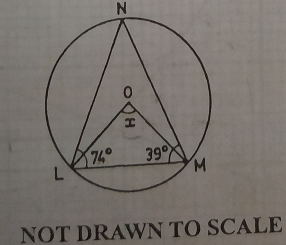mycollegehive
In the diagram, O is the centre of the circle. If ∠NLM = 74o, ∠LMN = 39o and ∠LOM = x, find the value of x
waec-2020 ssce-exam-2020 waec-mathematics-2020 math0In the diagram, O is the centre of the circle. If ∠NLM = 74o, ∠LMN = 39o and ∠LOM = x, find the value of x

A. 134o

B. 126o

C. 113o

D. 106o

266 viewsShareFollowUniversity of Lagos Nigeria
20 August 2020School not set Nigeria
20 August 20200LNM is a triangle and the sum of all interior angles of a triangle is 180.

74 + 39 + ∠LNM = 180

113 + ∠LNM = 180

∠LNM = 180 - 113

∠LNM = 67

x = 2 * ∠LNM

x = 2 * 67

x = 134Share### Related Tags

waec-2020

8 followers

273 questionsssce-exam-2020

6 followers

273 questionswaec-mathematics-2020

15 followers

50 questions